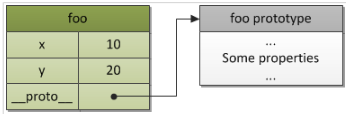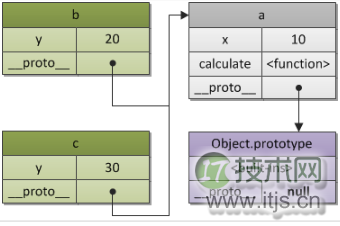﻿ JavaScript之原型深入详解_Javascript_IT技术网 ﻿

# JavaScript之原型深入详解

## 理解原型

```var foo = {
x: 10,
y: 20
};``````var a = {
x: 10,
calculate: function (z) {
return this.x + this.y + z
}
};
var b = {
y: 20,
__proto__: a
};

var c = {
y: 30,
__proto__: a
};

// call the inherited method
b.calculate(30); // 60```## 使用原型

```var decimalDigits = 2,
tax = 5;

return x + y;
}

function subtract(x, y) {
return x - y;
}

```var Calculator = function (decimalDigits, tax) {
this.decimalDigits = decimalDigits;
this.tax = tax;
};```

```Calculator.prototype = {
return x + y;
},

subtract: function (x, y) {
return x - y;
}
};

```var BaseCalculator = function() {
this.decimalDigits = 2;
};

BaseCalculator.prototype = {
return x + y;
},
subtract: function(x, y) {
return x - y;
}
};```

```var Calculator = function () {
//为每个实例都声明一个税收数字
this.tax = 5;
};

Calculator.prototype = new BaseCalculator();```

```var Calculator = function () {
this.tax= 5;
};

Calculator.prototype = BaseCalculator.prototype;```

```//覆盖前面Calculator的add() function
Calculator.prototype.add = function (x, y) {
return x + y + this.tax;
};

var calc = new Calculator();

## 原型链

```function foo() {
this.add = function (x, y) {
return x + y;
}
}

foo.prototype.add = function (x, y) {
return x + y + 10;
}

Object.prototype.subtract = function (x, y) {
return x - y;
}

var f = new foo();

```// 修改Object.prototype
Object.prototype.bar = 1;
var foo = {goo: undefined};

foo.bar; // 1
'bar' in foo; // true

foo.hasOwnProperty('bar'); // false
foo.hasOwnProperty('goo'); // true```

`alert(Cat.prototype.isPrototypeOf(cat2)); //true`

﻿